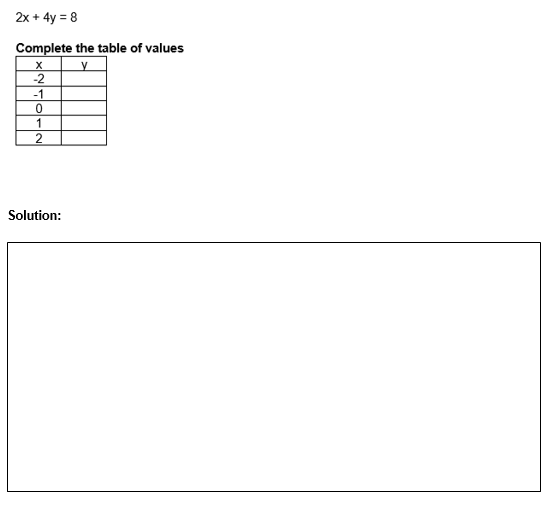# Table of Values Worksheet (with answer key + PDF)

The relationship between the various data points is displayed using tables of values. In science class, a table of values might be used. Tables of values are used by scientists and researchers to record their data, which is then examined for patterns. Then, they can use this pattern to predict outcomes. For instance, a table of values can be used by geologists to track the amount of underground movement in relation to the separation from a fault line.

## What is the “Table of Values Worksheet (with answer key + PDF)”?

This worksheet will explore some of the tables of values on how it’s function.

## What are tables of values?

A table of values is a collection of ordered pairs that are typically produced by replacing numbers in an equation (relation). The relationship between each pair of numbers in each ordered pair in the values table is determined by the equation.

This worksheet will help the student to solve and complete the table of values. Using this worksheet their understanding of the topic will be concrete and enable them to solve the given exercises below.

Instructions on how to use the “Table of Values Worksheet (with answer key + PDF).”

Examine the concept and examples provided, then attempt to complete the exercises below.

## Conclusion

To display the relationship between data items, tables of values are used. In science class, you might employ a table of values. Researchers and scientists record their data using tables of values, then examine the data to look for patterns. This pattern can then be applied to generate predictions.

If you have any inquiries or feedback, please let us know.

## Table of Values Worksheet (with answer key + PDF)

You can create a table of values from a linear equation by substituting values for x into the

equation and solving for y.

Complete the table of values for the equation.## Worksheet

Complete the table and show your solution.